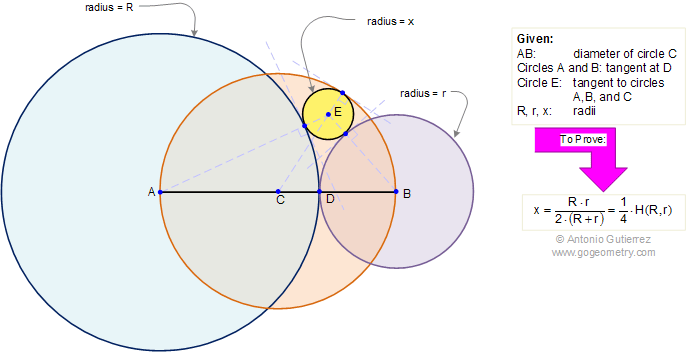Problem 288. Tangent circles, Harmonic Mean, Radius, Diameter

 In the figure below, AB is the diameter of a circle C, circles A (radius R) and B (radius r) are tangent at D. Circle E is tangent to circles A, B, and C. Prove that the radius x of circle E is one-fourth the harmonic mean of R and r. View or post a solution.Home | Sitemap | Geometry | Search | Problems | All | 281-290 | Semicircle | Harmonic Mean | Email | View or post a solution